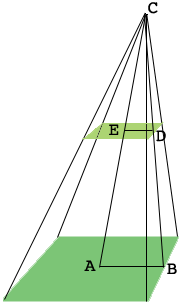SEARCH HOMEMath Central Quandaries & QueriesQuestion from Abdulganiy: A right pyramid on a base 10cm square is 15m high a)find the volume of the pyramid b)if the top 6m of the pyramid is removed what is the volume of the remaining frustum?Hi Abdulganiy,

The volume of a pyramid is given by

$\frac{1}{3} \times \mbox{ (the area of the base)} \times \mbox{ (the height)}$The volume you want is the difference between the volume of the large pyramid with a dark green base and the volume of the small pyramid with a light green base. The only fact you are missing is the size of the small green square. I suggest that you use the fact that triangles $ABC$ and $EDC$ are similar.

PennyMath Central is supported by the University of Regina and The Pacific Institute for the Mathematical Sciences.